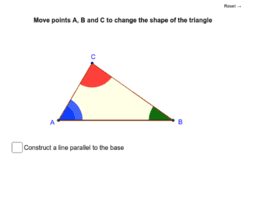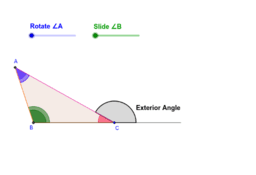# Lesson 9 - Angle Theorems of a Triangle

Author:
Mr. Coffie
This book contains GeoGebra pages that explore the theorems discussed in 10th grade geometry: the triangle angle-sum theorem and the triangle exterior angle-sum theorem.
•### Sum of the Angles in a Triangle

•